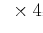Elastic wave-mode separation for TTI mediaNext: S wave-mode amplitudes Up: Discussion Previous: Discussion

## Computational issues

The separation of wave-modes for heterogeneous TI models requires non-stationary spatial filtering with large operators (operators ofsamples in each dimension are used in this chapter), which is computationally expensive. The cost is directly proportional to the size of the model and to the size of each operator. Furthermore, in a simple implementation, the storage for the separation operators of the entire model is proportional to the size of the model and to the size of each operator. Suppose that a 3D elastic TTI model is characterized by the model parameters,, Thomsen parametersand, and symmetry axis tilt angleand azimuth angle. For a 3D model ofgrid points, if one assumes that all operators have a size ofsamples, the storage for the operators isgrid pointssamples/independent operatorindependent operators/grid pointBytes/sampleTB. This is not feasible in ordinary processing. However, since there are relatively few medium parameters, i.e.,theratio,,, and anglesand, which determine the properties of the operators, one can construct a look-up table of operators as a function of these parameters, and search the appropriate operators at every location in the model when doing wave-mode separation. For example, suppose one knows that,,, and the symmetry axis tilt angleand azimuth angle, one can sample theratio at every,andat every, and the angles at every. In this case, one only needs a storage ofcombinations of medium parameterssample/independent operatorindependent operators/combination of medium parametersBytes/sampleGB; this is more manageable, although it is still a large volume to store.Elastic wave-mode separation for TTI mediaNext: S wave-mode amplitudes Up: Discussion Previous: Discussion

2013-08-29